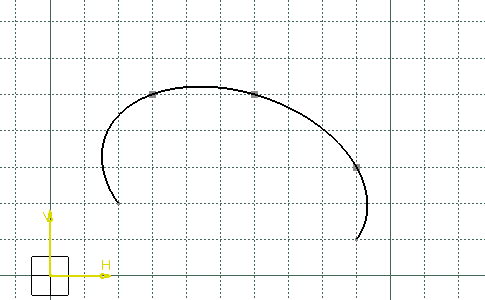# Creating Conic CurvesThis task shows you the different methods you can apply to create conic curves which are either arcs of parabolas, hyperbolas or ellipses.
The Sketch tools toolbar displays options for defining the conic: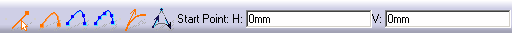• Conic creation type options are: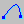Two Points type allows you to create a conic from two points (the start and end points), two tangencies at these points and either a parameter or a passing point. This type is activated by default. See Using Two Points and Start and End Tangent and Using Two Points and Tangent Intersection Point.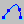Four Points type allows you to create a conic from four points (the start and end points, and two intermediate passing points) and one tangency at one of these points. Intermediates passing points have to be selected in logical order. See Using Four Points with a Tangency at Passing Point.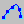Five Points type allows you to create a conic from five passing points (the start and end points, and three intermediate passing points). You cannot define a tangency at any of these point. Intermediates passing points have to be selected in logical order. See Using Five Points.
• Conic creation mode options are: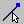Nearest End Point allows you to create a conic based on existing curved. If the selected points belong to a curve the tangent direction is directly read on the curve. This mode is activated by default. See Using Two Points with the Nearest End Point Mode.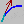Start and End Tangent mode allows you to define: The tangencies at start and end points for Two Points type. For Two Points type, this mode is activated by default, see Using Two Points and Start and End Tangent. The tangency at one point only for Four Points type, if you deactivate this mode for the three first created points, a tangency must be automatically defined for the last point. Each time you redefine a tangency at one point, the previous defined tangency is removed, see Using Four Points with a Tangency at Passing Point. For Four Points type, this mode is activated by default. This mode is available with Two Points and Four Points types only, and for these types activated by default.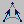Tangent Intersection Point mode, available with Two Points type only, allows you to define the intersection point of the start and end tangents. The start and end tangents are defined from this point and the start and end points respectively. This mode deactivates the Start and End Tangent mode. See Using Two Points and Tangent Intersection Point.

• The conic is variational and associative with the geometrical inputs, which means that it will be updated after every modification of a geometry input.
• You can also edit the curve or add constraints to it.
• You cannot trim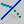nor break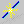conic curves. To workaround this restriction, you can project the conic curve of interest onto another sketch, then trim the projected elements.

## Using Two Points and Start and End Tangent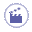1. Click Conic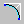.

2. Select the Two Points type.

3. Click to indicate the start point: H=20mm, V=20mm.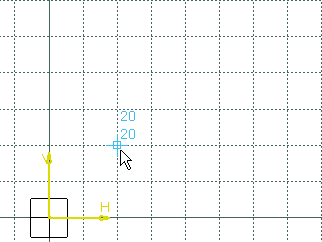1. Type in the Sketcher tools toolbar for the start tangent point: H=30mm, V=50mm and press Enter.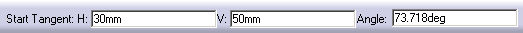The start point and tangent have been created.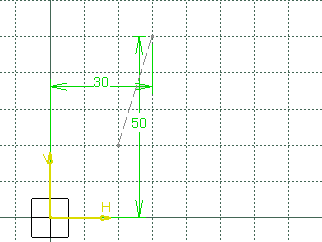1. Click to indicate the end point: H=90mm, V=10mm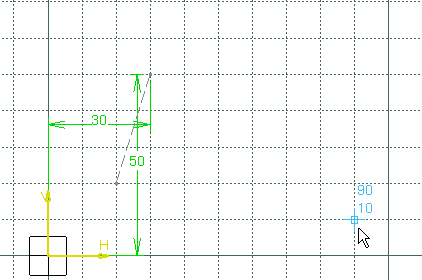1. Type in the Sketcher tools toolbar for the end tangent point: Angle=120deg, and H=70mm, and press Enter.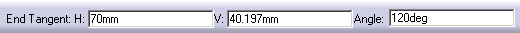### With a Parameter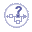The parameter value is a ratio ranging from 0 to 1 (excluded), this value is used to define a passing point:

• If parameter = 0.5, the resulting curve is a parabola.

• If 0 < parameter < 0.5, the resulting curve is an arc of ellipse.

• If 0.5 < parameter < 1, the resulting curve is a hyperbola.

1. Type in the Sketcher tools toolbar for the parameter: Parameter=0.3 and press Enter.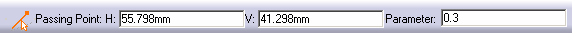The conic is created.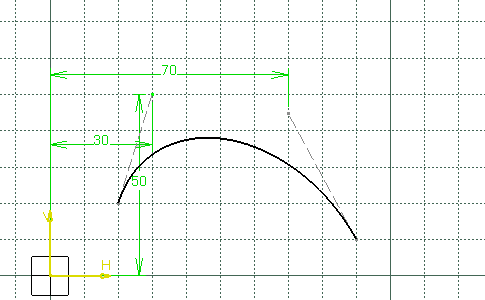### With a Passing Point

1. Type in the Sketcher tools toolbar for the parameter: H=50mm, V=40mm and press Enter.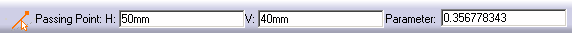The conic is created.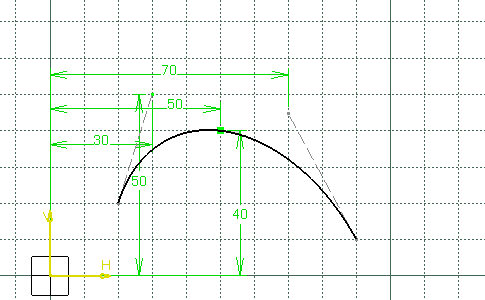## Using Two Points and Tangent Intersection Point1. Click Conic.

1. Select the Two Points type.

1. Select Tangent Intersection Point.

1. Click to indicate the start point:  H=20mm, V=20mm1. Click to indicate the end point: H=90mm, V=10mm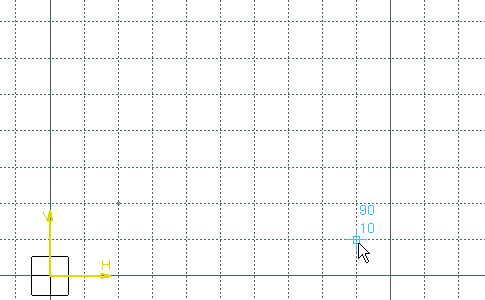1. Click to indicate the tangent intersection point: H=60mm, V=60mm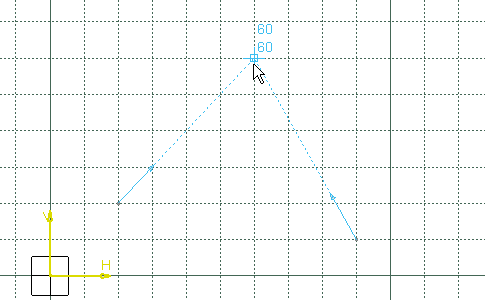### With a ParameterThe parameter value is a ratio ranging from 0 to 1 (excluded), this value is used to define a passing point:

• If parameter = 0.5, the resulting curve is a parabola.

• If 0 < parameter < 0.5, the resulting curve is an arc of ellipse.

• If 0.5 < parameter < 1, the resulting curve is a hyperbola.

1. Type in the Sketcher tools toolbar for the parameter: Parameter=0.3 and press Enter.The conic is created.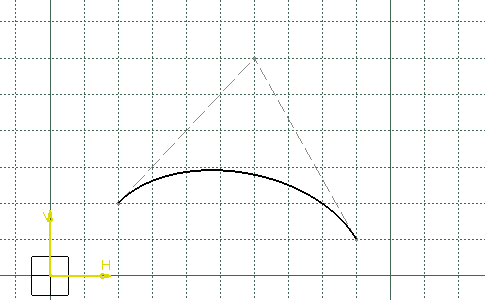### With a Passing Point

1. Click to indicate the passing point: H=50mm, V=40mm.
The conic is created.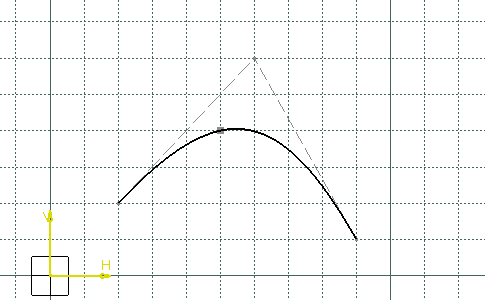## Using Two Points with the Nearest End Point Mode1. Create two lines, the first between H=10mm, V=0mm and H=40mm, V=60mm, and the second between H=90mm, V=0mm and H=90mm, V=40mm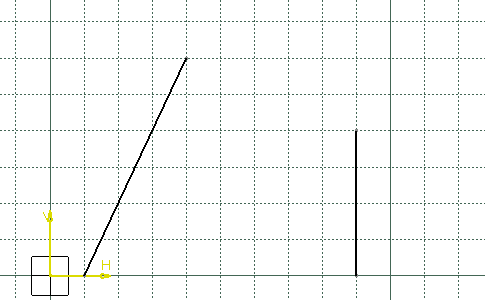1. Click Conic.

1. Select the Two Points type.

1. Select the Nearest End Point mode.

1. Click to indicate the start point: H=20mm, V=20mm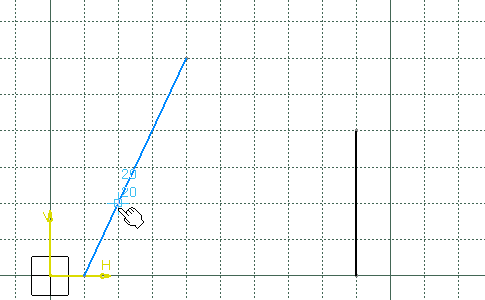1. Click to indicate the end point: H=90mm, V=10mm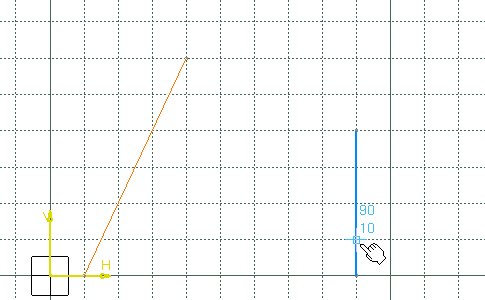1. Click to indicate the passing point: H=60mm, V=40mm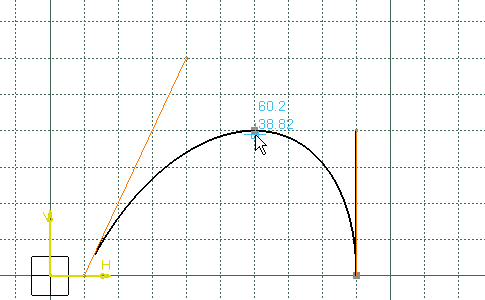The conic is created:

• The tangents at the start and end points have been defined by the lines.

• The start and end points taken into account are the nearest extremities of the lines during the selection.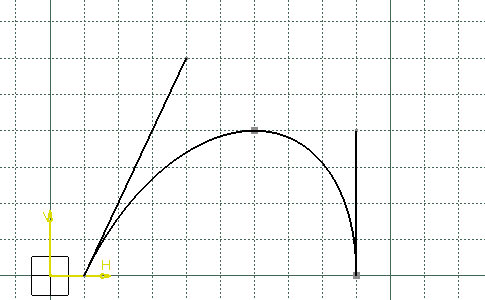When you redo the previous steps deactivating the Nearest End Point mode:

• The tangents at the start and end points have been defined by the lines.

• The start and end points taken into account are the selected points on the lines.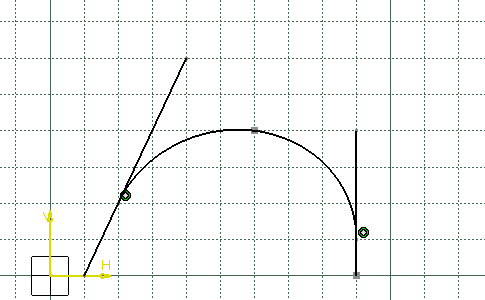## Using Four Points with a Tangency at Passing Point1. Click Conic.

1. Select the Four Points type.

1. Click to indicate the start point: H=20mm, V=20mm1. Type in the Sketcher tools toolbar for the start tangent point: H=30mm, V=50mm and press Enter.The start point and tangent have been created.1. Click to indicate the end point: H=90mm, V=10mm1. Select the Start and End Tangent mode.

1. Click to indicate the first passing point: H=60mm, V=50mm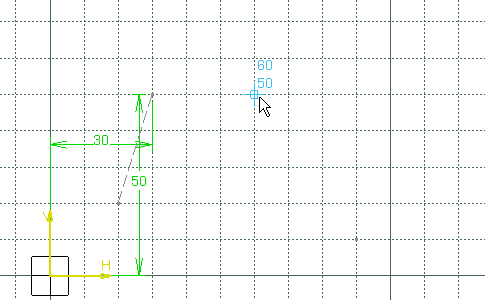1. Type in the Sketcher tools toolbar for the first tangent point: H=90mm, V=50mm and press Enter.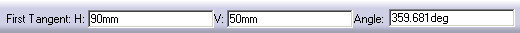1. Click to indicate the second passing point: H=100mm, V=40mm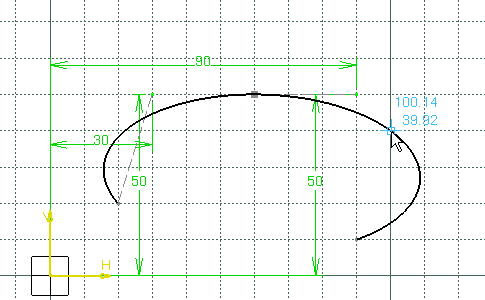The conic is created. The defined tangent at the start point has been released and the construction line representing the tangent has been removed.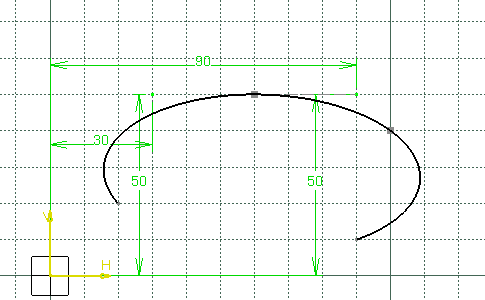## Using Five Points1. Click Conic.

1. Select the Five Points type.

1. Click to indicate the start point: H=20mm, V=20mm1. Click to indicate the end point: H=90mm, V=10mm1. Click to indicate the first passing point: H=30mm, V=50mm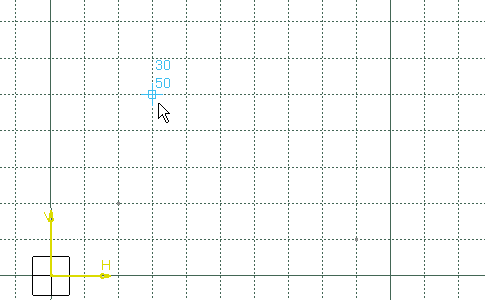1. Click to indicate the second passing point: H=60mm, V=50mm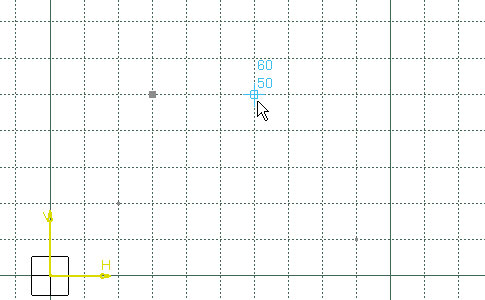1. Click to indicate the third passing point: H=90mm, V=30mm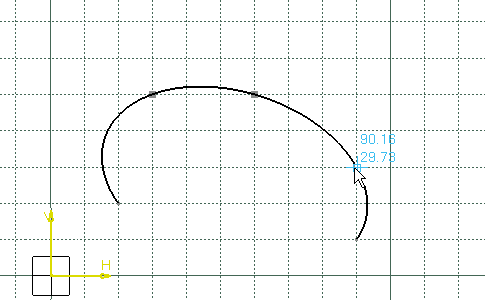The conic is created.